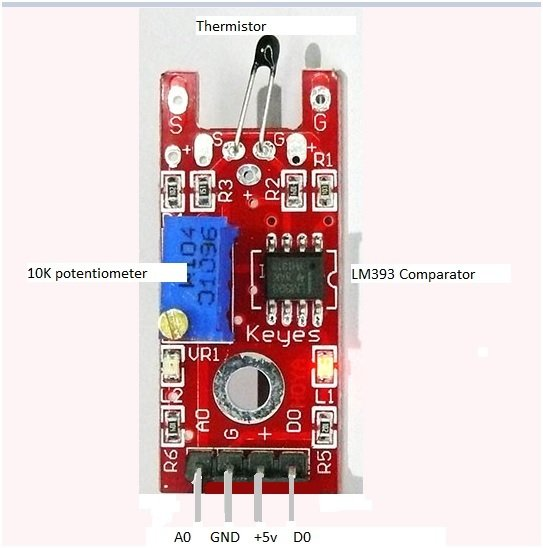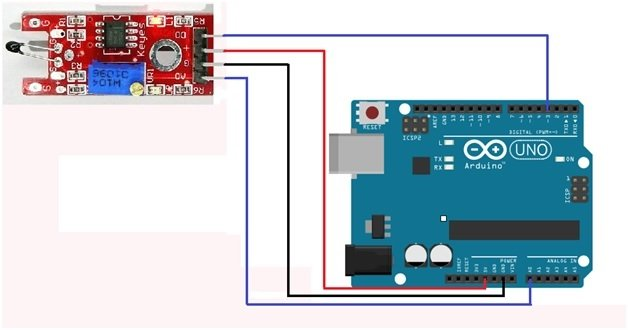# thermistor interfacing with arduino temperature meter

Thermistor interfacing with Arduino: This module is used to measure the temperature and it gives you output both at analog and digital pin. This module has many components like thermistor, 100k ohm potentiometer, and lm393 comparator.

• Thermistor: Thermistor is a very cheap, inexpensive and accurate sensor. It is a like a variable resistor whose resistance changes with the change in temperature.
• 100k ohm Potentiometer: It is basically used for the digital output. The digital output can be changed by changing the value of potentiometer
• LM393 comparator: The lm393 comparator will compare the value of thermistor and the potentiometer and will give the digital output according to it.

The module has both analog and digital output

• The analog output will give you a varying voltage depending upon the temperature applied to it.
• The digital output will give you 1 or 0 logic set by the blue component on the board which is potentiometer.

### How thermistor works?

The value of the thermistor changes with the change in temperature. A 100k ohm variable resistor is connected in series with the thermistor across +5v and ground. The center pin of the potentiometer has a voltage that changes with temperature. The analog output can be taken from the A0 of module and it will be measured by Arduino. . For the digital output, an lm393 comparator will compare the voltage from the thermistor and from the potentiometer and will give output in logic 1 and 0 at the D0.

### Pin out of thermistor moduleThe module has four pins

A0: For analog output

D0: For digital output

Gnd: Connect it to ground

5V: For power

### Thermistor module interfacing with arduinoThe connections are very simpler. Connect the 5v and the ground of the module to the 5v and ground of the Arduino and the analog and digital pins to A0 and pin 3 of Arduino. If you want to increase the set point for the digital output then move the top of the potentiometer with the screw. If the led is off then turn the top clockwise until led becomes on then move in other direction until it gets off.

### Code of thermistor module interfacing with arduino

Code for thermistor interfacing with arduino is given below. Comments are made with each line for user understanding and every line is very clear and understandable.

```// This code is for the Analog and digital temperature sensor module

#define LED_PIN 13                                          // defining pin 13 as the led input pin

#define DIGITAL_INPUT 3                              // defining digital input at pin 3

// initializing the variables

int    digital_output ;                                                        // This will read the digital value

int    analog_output ;                                                       // This will read the analog value

int    revised_output;                                                      // variable to store the corrected value

float  temp_C ;                                                                  // Variable for storing the temperature

float  temp_f ;                                                                   // Variable for storing the fahrenheit

void setup ( )                                                                     // Anything written I it will run once.

{

pinMode ( LED_PIN, OUTPUT ) ;                               // declaring pin 13 as output

pinMode ( DIGITAL_INPUT, INPUT ) ;                   // declaring pin 3 as input

pinMode ( ANALOG_INPUT, INPUT )  ;                 // declaring A0 as input pin

Serial.begin ( 9600 ) ;                                                     // selecting the baud rate at 9600

Serial.println (" microcontrollerslab.com : The data is " ) ;

}

void loop ( )                                                                                        // Anything written in it will run continuously

{

analog_output = analogRead ( ANALOG_INPUT ) ;         // Reading the analog value and storing in analog_output

Serial.print ( " Analog value of the module is =  " ) ;

Serial.print ( analog_output ) ,  DEC ;                                    // This will display the analog value

// The module has thermistor connection reversed

revised_output= map ( analog_output, 0, 1023, 1023, 0 ) ;

temp_C    = Thermistor ( revised_output ) ;

temp_f = ( temp_C * 9.0 ) / 5.0 + 32.0 ;

digital_output = digitalRead ( DIGITAL_INPUT ) ;

Serial.print ( "   Digital value of the module is =  " ) ;

Serial.println ( digital_output ) , DEC ;                                   // This will display the digital value on the display

Serial.print ( " LED is =" ) ;

if ( digital_output == HIGH )                      // The LED will turn on When the sensor value will exceed the set point

{

digitalWrite ( LED_PIN, HIGH ) ;

Serial.print ( "ON " ) ;

}

else

{

digitalWrite ( LED_PIN, LOW ) ;

Serial.print ( "OFF " ) ;

}

// This will print the temperature

Serial.print ( " Measured Temperature = " ) ;

Serial.print ( temp_f, 1 ) ;                                           // This will display the temperature in Fahrenheit

Serial.print (" F  " ) ;

Serial.print  (temp_C, 1 ) ;                                          // This will display the temperature in Celcius

Serial.println (" C " ) ;

Serial.println ( ) ;                                                            // Leaving a blank line

Delay ( 1000 ) ;                                                                   // Wait for 1 second

}

double Thermistor ( int RawADC )

{

double Temp ;

Temp = log ( ( ( 10240000 / RawADC ) – 10000 ) ) ;

Temp = 1 / ( 0.001129148 + ( 0.000234125 * Temp ) + ( 0.0000000876741 * Temp * Temp * Temp ) ) ;

Temp = Temp - 273.15 ;            // This will Convert Kelvin to Celcius

return Temp ;

}```
```The output will look like this

microcontrollerslab.com : The data is

Analog value of the module is = 768   Digital value of the module is = 1

LED is = OFF Measured Temperature = 80.2 F 19.3 C```